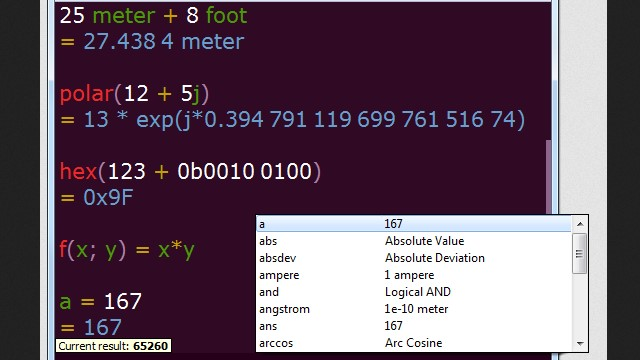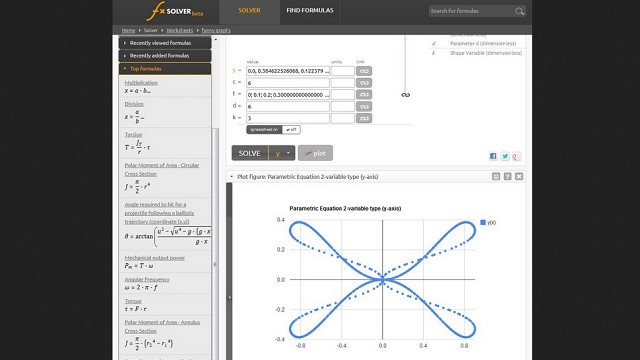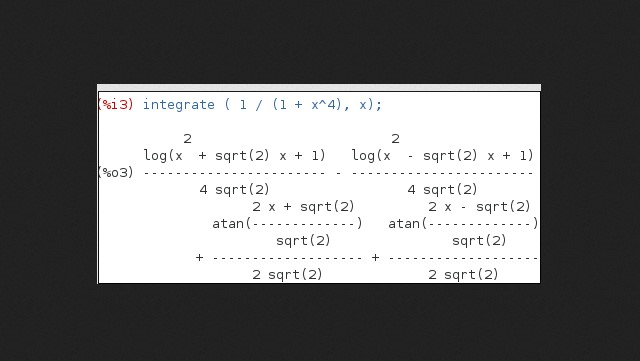# Mathway AlternativeMath is one of the subjects that many students have problems with. Some students even are scared of this subject. The internet has developed many methods to help students to learn math easily. One of them is Mathway.

It is an online tool that students can use to solve Math problems. Moreover, it also shows the steps-by-steps of the solution to those problems. However, Mathway is not the only tool for understanding Math available today. There are many programs as Mathway Alternative you can find out there.

## The Best Mathway Alternative You Can Use

### 1. GeoGebra Apps

One of the best Math software as an alternative to Mathway is GeoGebra. It uses a dynamic process to provide the solution for the Math problem. This software has all solutions for algebra, geometry, graphing, spreadsheets questions, calculus, and statistics. All of them are in one easy-to-use software. Many schools that use STEM curriculum also recommend this software as the supporting tool.#### GeoGebra Apps Features

• Computations with fractions
• Trigonometric functions: sin, cos, tan
• Statistics functions
• Exponential functions and logarithms
• Plot functions, implicit equations, polar and parametric curves
• Find derivatives and integrals.
• Experience transformations with sliders
• Find special points of functions: roots, min, max, intersections
• Construct and analyze many types of regressions.
• Create geometric constructions
• Measure lengths, perimeters, and areas
• Create and analyze traces of points and loci
• Plot functions, polar and parametric curves
• Experience transformations with sliders
• Get special points of functions: roots, min, max, intersections
• Do regression with best-fit lines
• Save and share your results with friends and teachers

### 2. SageMath

SageMath is a combination of several open-source Math software. You might recognize some of them, such as NumPy and matpoltib. This unique building allows you to write the question and solve it much easier. It also has a screenshot feature that you can use to capture the solution as the study material or a tool to teach the student.#### SageMath Features

• SageMath covering many aspects of mathematics
• Including algebra
• Combinatorics
• Graph theory
• Numerical analysis
• Number theory
• Calculus and statistics

### 3. SpeedCrunch

SpeedCrunch is a lightweight software that won’t use too many resources of your device. It is easy to install and use, which is a perfect choice for students. Moreover, it doesn’t limit its feature on syntax shape. You can write the Math problem on it, and it will be able to recognize it without fail. With hundreds of mathematical functions and scientific constant in it, it will solve any Math problem you put in it.#### SpeedCrunch Features

• Free and Open-source software
• Efficient & easy-to-use interface
• SpeedCrunch comes with over 80 built-in mathematical functions
• A built-in formula book allows you to review
• Cross-platform
• And multi-language

### 4. fxSolver

fxSolver is software made for users of any level. Beginner or professional can use it to formulate any Math problem and find its solution in the simplest process. It can act as a virtual assistant for learning Math. Its editable interface also allows you to not only solve the problem but also create the Math problem that other students can try to solve.#### fxSolver Features

• Equation library
• Graphing calculator
• Science and engineering problem helper
• Calculation of multiple equations at once
• Formula editor

### 5. Maxima

Maxima is one of the complete tools for solving the Math problem. Moreover, it is an open-source program that allows you to configure it as much as you want through GNU Emacs mode. This program also has one of the best features to recognize and manipulate all kinds of symbols and expressions in Math. Therefore, it is a perfect tool for any Math level.#### Maxima Features

• Specialized in symbolic operations but offering numerical capabilities too
• Can be accessed programmatically and extended, as the underlying Lisp can be called from it
• A complete programming language with ALGOL-like syntax but Lisp-like semantics
• Arbitrary-precision integers
• Rational numbers of sizes limited only by machine memory
• Arbitrarily large floating-point numbers

### 6. MathType

MathType focuses on providing a tool to let you type and create Math functions, equations, formulas, or problems with ease. It has an interactive appearance and simple design that allow you to input any Math sign and symbol easier.#### MathType Features

• Publication-quality equations
• Integration in Microsoft Word and other apps
• Compatibility with software and standards
• Productivity
• Customization
• Styling and fonts your choice
• Complete coverage for all levels
• Easy installation

### 7. Photomath

Photomath is the best math solving app, better than the competition. The app has never gotten an answer incorrect, it is also easy to use and very simple. Photomath has been helpful in many ways, it has some different categories, it has an option to search some things so you can search faster. I totally recommend this app to everyone.#### Photomath Features

• Word problem explanations
• No internet or data required for equation-based problems, Free to use
• Step-by-step explanations for every solution
• Exclusive how-to animations
• Scroll through multiple solving methods per problem
• Multi-functional scientific calculator
• Interactive graphs

### 8. Microsoft Math Solver

This app is a great tool to help you complete your work faster. It easily helps you find answers to the math problems and makes it easy to do classwork. Microsoft Math Solver is a major help when it comes to Trinomals, and Factor by grouping, etc. It gives the correct answer, it does let you see all the steps without paying.#### Microsoft Math Solver Features

• Write an equation on screen as you naturally do on paper
• Scan printed or handwritten math problem
• Type and edit using an advanced scientific calculator
• Get interactive Step-by-Step explanations & Graphing calculator
• Import images with equations from the gallery
• Scan and Solve Worksheets with multiple problems
• Search the web for similar problems and video lectures
• Try word problems
• Scan and plot x-y data tables for linear/non-linear functions
• Learn mathematics in your language – supports Chinese, French, German, Hindi, Italian, Japanese, Portuguese, Russian, Spanish, and many more

### Conclusion

Those are some programs that you can use as Mathway Alternative. They are good for solving a Math problem and easy to use. So, if you think Mathway is not your tool, try to use one of those tools. You will get what you need from them.